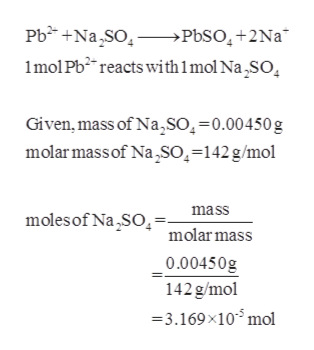# The concentration of lead ions (Pb2+) in a sample of polluted water that also contains nitrate ions (NO3−) is determined by adding solid sodium sulfate (Na2SO4) to exactly 500 mL of the water. Calculate the molar concentration of Pb2+ if 0.00450 g of Na2SO4 was needed for the complete precipitation of lead ions as PbSO4.

Question
28 views

The concentration of lead ions (Pb2+) in a sample of polluted water that also contains nitrate ions (NO3) is determined by adding solid sodium sulfate (Na2SO4) to exactly 500 mL of the water. Calculate the molar concentration of Pb2+ if 0.00450 g of Na2SO4 was needed for the complete precipitation of lead ions as PbSO4.

check_circle

Step 1

The balanced reaction of lead ions with...help_outlineImage TranscriptionclosePbNa So >PbSO4+2Na* 1mol Pb2 reacts with1mol Na SO Given, mass of Na,so,=0.00450 g molarmassof Na SO=142g/mol mass moles of Na SO molar mass 0.00450g 142g/mol =3.169x10 mol fullscreen

### Want to see the full answer?

See Solution

#### Want to see this answer and more?

Solutions are written by subject experts who are available 24/7. Questions are typically answered within 1 hour.*

See Solution
*Response times may vary by subject and question.
Tagged in

### Physical Chemistry